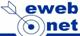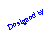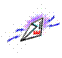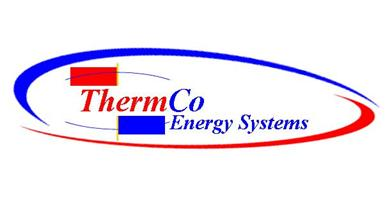Retro Fitting is thinking   GREEN – RECYCLING          REDUCING CARBON FOOTPRINTConverting KW / TON to COP or EER
 Example: if equipments efficiency is rated at 1 KW/ton, the COP=3.5 and the EER=12
 Calculate  Electric Utilization Index (EUI) by dividing annual KWh consumption by the facility's square footage.(ex: If a chiller's efficiency is rated at 1 KW/ton, the COP=3.5 and the EER=12) KW/ton = (12)/EER KW/ton = (12)/(COP x 3.412) EER = (12)/KW/ton EER = COP x 3.412 COP = (EER)/3.412 COP = 12/(KW/ton)/3.412